Thank you for visiting nature.com. You are using a browser version with limited support for CSS. To obtain the best experience, we recommend you use a more up to date browser (or turn off compatibility mode in Internet Explorer). In the meantime, to ensure continued support, we are displaying the site without styles and JavaScript.

# Suppressing qubit dephasing using real-time Hamiltonian estimation

## Abstract

Unwanted interaction between a quantum system and its fluctuating environment leads to decoherence and is the primary obstacle to establishing a scalable quantum information processing architecture. Strategies such as environmental and materials engineering, quantum error correction and dynamical decoupling can mitigate decoherence, but generally increase experimental complexity. Here we improve coherence in a qubit using real-time Hamiltonian parameter estimation. Using a rapidly converging Bayesian approach, we precisely measure the splitting in a singlet-triplet spin qubit faster than the surrounding nuclear bath fluctuates. We continuously adjust qubit control parameters based on this information, thereby improving the inhomogenously broadened coherence time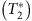from tens of nanoseconds to >2 μs. Because the technique demonstrated here is compatible with arbitrary qubit operations, it is a natural complement to quantum error correction and can be used to improve the performance of a wide variety of qubits in both meteorological and quantum information processing applications.

## Introduction

Hamiltonian parameter estimation is a rich field of active experimental and theoretical research that enables precise characterization and control of quantum systems1. For example, magnetometry schemes employing Hamiltonian learning have demonstrated dynamic range and sensitivities exceeding those of standard methods2,3. Such applications focused on estimating parameters that are quasistatic on experimental timescales. However, the effectiveness of Hamiltonian learning also offers exciting prospects for estimating fluctuating parameters responsible for decoherence in quantum systems.

The quantum system that we study is a singlet-triplet (ST0) qubit4,5 which is formed by two gate-defined lateral quantum dots (QDs) in a GaAs/AlGaAs heterostructure (Fig. 1a, Supplementary Fig. 1), similar to that of refs 6, 7. The qubit can be rapidly initialized in the singlet state |S› in ≈20 ns and read out with 98% fidelity in ≈1 μs (refs 8, 9; Supplementary Fig. 2). Universal quantum control is provided by two distinct drives10: the exchange splitting, J, between |S› and |T0›, and the magnetic field gradient, ΔBz, due to the hyperfine interaction with host Ga and As nuclei. The Bloch sphere representation for this qubit can be seen in Fig. 1b. In this work, we focus on qubit evolution around ΔBz (Fig. 2a). Due to statistical fluctuations of the nuclei, ΔBz varies randomly in time, and consequently oscillations around this field gradient decay in a time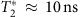(ref. 4). A nuclear feedback scheme relying on dynamic nuclear polarization11 can be employed to set the mean gradient, (g*μBΔBz/h≈60 MHz in this work) as well as reduce the variance of the fluctuations. Here, g*≈−0.44 is the effective gyromagnetic ratio in GaAs, μB is the Bohr magneton and h is Planck’s constant. In what follows, we adopt units where g*μB/h=1. The nuclear feedback relies on the avoided crossing between the |S› and |T+› states. When the electrons are brought adiabatically through this crossing, their total spin changes by Δms=±1, which is accompanied by a nuclear spin flip to conserve angular momentum. With the use of this feedback, the coherence time improves to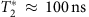(ref. 11; Fig. 2b), limited by the low nuclear pumping efficiency10. Crucially, the residual fluctuations are considerably slower than the timescale of qubit operations12.

In this work we employ techniques from Hamiltonian estimation to prolong the coherence of a qubit by more than a factor of 30. Importantly, our estimation protocol, which is based on recent theoretical work13, requires relatively few measurements (≈100) which we perform rapidly enough (total time ≈100 μs) to resolve the qubit splitting faster than its characteristic fluctuation time. We adopt a paradigm in which we separate experiments into ‘estimation’ and ‘operation’ segments, and we use information from the former to optimize control parameters for the latter in real-time. Our method dramatically prolongs coherence without using complex pulse sequences such as those required for non-identity dynamically decoupled operations14.

## Results

### Rotating frame S−T0 qubit

To take advantage of the slow nuclear dynamics, we introduce a method that measures the fluctuations and manipulates the qubit on the basis of precise knowledge but not precise control of the environment. We operate the qubit in the rotating frame of ΔBz, where qubit rotations are driven by modulating J at the frequency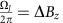(refs 15, 16). This is in contrast to traditional modes of operation of the ST0 qubit, which rely on DC voltage pulses. To measure Rabi oscillations, the qubit is adiabatically prepared in the ground state of ΔBz (|ψ›=|↑↓›), and an oscillating J is switched on (Fig. 2e), causing the qubit to precess around J in the rotating frame. In addition, we perform a Ramsey experiment (Fig. 2c) to determine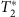, and as expected, we observe the same decay (Fig. 2d) as Fig. 2b. More precisely, the data in Fig. 2d represent the average of 1,024 experimental repetitions of the same qubit operation sequence immediately following nuclear feedback. The feedback cycle resets ΔBz to its mean value (60 MHz) with residual fluctuations of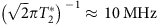between experimental repetitions. However, within a given experimental repetition, ΔBz is approximately constant. Therefore we present an adaptive control scheme where, following nuclear feedback, we quickly estimate ΔBz and tuneto prolong qubit coherence (Fig. 3a).

### Bayesian estimation

To estimate ΔBz , we repeatedly perform a series of single-shot measurements after allowing the qubit to evolve around ΔBz (using DC pulses) for some amount of time (Fig. 2a). Rather than fixing this evolution time to be constant for all trials, we make use of recent theoretical results in Hamiltonian parameter estimation13,16,17 and choose linearly increasing evolution times, tk=ktsamp, where k=1,2,…N. We choose the sampling time tsamp such that the estimation bandwidth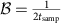is several times larger than the magnitude of the residual fluctuations in ΔBz, roughly 10 MHz. With a Bayesian approach to estimate ΔBz in real-time, the longer evolution times (large k) leverage the increased precision obtained from earlier measurements to provide improved sensitivity, allowing the estimate to outperform the standard limit associated with repeating measurements at a single evolution time. Denoting the outcome of the kth measurement as mk (either |S› or |T0›), we define P(mkBz) as the conditional probability for mk given a value ΔBz. We write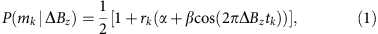where rk=1 (−1) for mk=|S›(|T0›), and α=0.25 and β=0.67 are parameters determined by the measurement error and axis of rotation on the Bloch sphere (see Methods). Since we assume that earlier measurement outcomes do not affect later ones (that is, that there is no measurement back-action), we write the conditional probability for ΔBz given the results of N measurements as: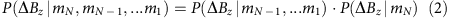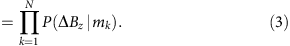Using Bayes’ rule, that is, PBz|mk)=P(mkBz)PBz)/P(mk), and equation (1), we can rewrite equation (3) as: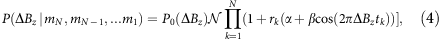where N is a normalization constant and P0Bz) is a prior distribution to which the algorithm is empirically insensitive, and which we take to be a constant over the estimation bandwidth. After the last measurement, we find the value of ΔBz that maximizes the posterior distribution PBz|mN,mN−1,...m1).

We implement this algorithm in real-time on a field-programmable gate array (FPGA), computing PBz) for 256 values of ΔBz between 50 and 70 MHz. With each measurement mk, the read-out signal is digitized and passed to the FPGA, which computes PBz) and updates an analogue voltage that tunes the frequency of a voltage controlled oscillator (Fig. 1a; Supplementary Note 1, Supplementary Fig. 3). Following the Nth sample,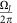nearly matches ΔBz, and since the nuclear dynamics are slow, the qubit can be operated with long coherence without any additional complexity. To quantify how well the FPGA estimate matches ΔBz, we perform a Ramsey experiment (deliberately detuned to observe oscillations) with this real-time tracking of ΔBz and find optimal performance for N≈120, with a maximum experimental repetition rate, limited by the FPGA, of 250 kHz and a sampling time tsamp=12 ns. Under these conditions, and making a new estimate after every 42 Ramsey experiments, we observe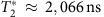, a 30-fold increase in coherence (Fig. 3b). We note that these data are taken with the same pulse sequence as those in Fig. 2d. To further compare qubit operations with and without this technique, we measure Ramsey fringes for ≈250 s (Fig. 3d), and histogram the observed Ramsey detunings. With adaptive control we observe a stark narrowing of the observed frequency distribution, consistent with this improved coherence (Fig. 3c).

## Discussion

Although the estimation scheme employed here is theoretically predicted to improve monotonically with N (ref. 13), we find that there is an optimum (N≈120), after whichslowly decreases with increasing N (Fig. 4a). A possible explanation for this trend is fluctuation of the nuclear gradient during the estimation period. To investigate this, we obtain time records of ΔBz using the Bayesian estimate and find that its variance increases linearly in time at the rate of (6.7±0.7 kHz)2 μs−1 (Fig. 4c). The observed linear behaviour suggests a model where the nuclear gradient diffuses, which can arise, for example, from dipolar coupling between adjacent nuclei. Using the measured diffusion of ΔBz, we simulate the performance of the Bayesian estimate as a function of N (see Methods). Given that the simulation has no free parameters, we find good agreement with the observed, indicating that indeed, diffusion limits the accuracy with which we can measure ΔBz (Fig. 4a).

This model suggests that increasing the rate of measurements during estimation will improve the accuracy of the Bayesian estimate. Because our FPGA limits the repetition rate of qubit operations to 250 kHz, we demonstrate the effect of faster measurements through software post-processing with the same Bayesian estimate. To do so, we first use the same estimation sequence, but for the operation segment, we measure the outcome after evolving around ΔBz for a single evolution time, tevo, rather than performing a rotating frame Ramsey experiment, and we repeat this experiment a total of Ntot times. In processing, we perform the Bayesian estimate of each ΔBz,i, sort the data by adjusted time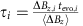(for i=1,2,…Ntot), and average together points of similar τ to observe oscillations (see Methods). We fit the decay of these oscillations to extractand the precision of the Bayesian estimate,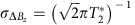. For the same operation and estimation parameters, we find thatextracted from software post-processing agrees with that extracted from adaptive control Supplementary Fig. 4, Supplementary Note 2. Using a repetition rate as high as 667 kHz, we show coherence times above 2,800 ns, corresponding to an error of σΔBz=80 kHz (Fig. 4d), indicating that improvements are easily attainable by using faster (commercially available) FPGAs.

In addition, we use this post-processing to examine the effect of this technique on the duty cycle of experiments as well as the stability of the ΔBz estimate. To do so we introduce a delay Tdelay between the estimation of ΔBz and the single evolution measurement performed in place of the operation. We find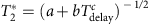, where c=0.99 (Fig. 4c), consistent with diffusion of ΔBz. Indeed, this dependence underscores the potential of adaptive control, since it demonstrates that after a single estimation sequence, the qubit can be operated for >1 ms with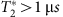. Thus, adaptive control need not significantly reduce the experimental duty cycle.

In this work, we have used real-time adaptive control on the basis of Hamiltonian parameter estimation of a ST0 spin qubit to prolongfrom 70 ns to >2 μs. Dephasing due to nuclear spins has long been considered a significant obstacle to quantum information processing using semiconductor spin qubits18, and elimination of nuclear spins is an active and fruitful area of research19,20,21. However, here we have shown that with a combination of nuclear feedback, rotating frame ST0 spin resonance, and real-time Hamiltonian estimation, we are able to achieve ratios of coherence times to operation times in excess of 200 without recourse to dynamical decoupling12,22,23. If the same adaptive control techniques were applied to gradients as high as 1 GHz (ref. 10), ratios exceeding 4,000 would be possible, and longer coherence times may be attainable with more sophisticated techniques13. Though the observed coherence times are still smaller than the Hahn echo time,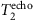(ref. 12), the method we have presented is straightforward to implement, compatible with arbitrary qubit operations, and general to all qubits that suffer from non-Markovian noise. Looking ahead, it is likely, therefore, to have a key role in realistic quantum error correction efforts24,25,26,27, where even modest improvements in baseline error rate greatly diminish experimental complexity and enhance prospects for a scalable quantum information processing architecture.

## Methods

### Bayesian estimate

We wish to calculate the probability that the nuclear magnetic field gradient has a certain value, ΔBz, given a particular measurement record comprising N measurements. We follow the technique in Sergeevich et al.13 with slight modifications. Writing the outcome of the kth measurement as mk, we write this probability distribution as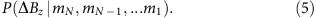To arrive at an expression for this distribution, we will write down a model for the dynamics of the system, that is, P(mN,mN−1,...m1Bz). Using Bayes’ rule we can relate the two equations as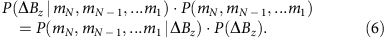First, we seek a model that can quantify P(mN,mN−1,...m1Bz) that accounts for realistic errors in the system, namely measurement error, imperfect state preparation and error in the axis of rotation around the Bloch sphere. For simplicity, we begin with a model that accounts only for measurement error. Denoting the error associated with measuring a |S› (|T0›) as ηS (ηT), we write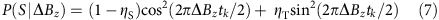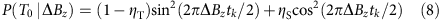We combine these two equations and write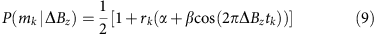where rk=1 (−1) for mk=|S›(|T0›) and α and β are given by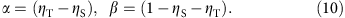Next, we generalize the model to include the effects of imperfect state preparation, and the presence of non-zero J during evolution, which renders the initial state non-orthogonal to the axis of rotation around the Bloch sphere (see above). We assume that the angle of rotation around the Bloch sphere lies somewhere in the xz plane and makes an angle θ with the z axis. We define δ=cos2 (θ). Next, we include imperfect state preparation by writing the density matrix ρinit=(1−ε)|S› ‹S|)+ε|T0› ‹T0|. With this in hand, we can write down the model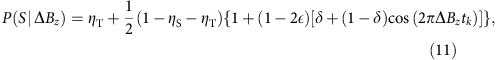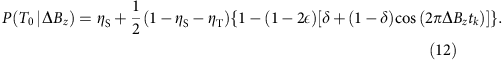Using the same notation for rk=1 (−1) for mk=|S›(|T0›), we rewrite this in one equation as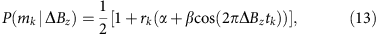where we now have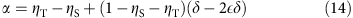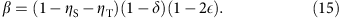We find the best performance for α=0.25 and β=0.67, which is consistent with known values for qubit errors.

We next turn our attention to implementing Bayes’ rule to turn this model into a probability distribution for ΔBz. First, we assume that all measurements are statistically independent, allowing us to write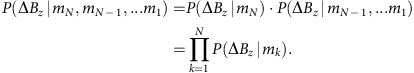We next use Bayes rule (6) and rewrite this equation as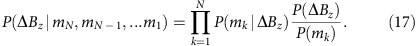Using our model (13) we can rewrite this as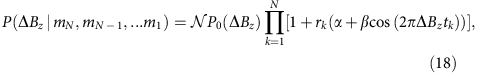where N is a normalization constant, and P0Bz) is a prior distribution for ΔBz which we take to be a constant over the estimation bandwidth, and to which the estimator is empirically insensitive. With this formula, it is simple to see that the posterior distribution for ΔBz can be updated in real time with each successive measurement. After the Nth measurement, we choose the value for ΔBz, which maximizes the posterior distribution (18).

### Simulation with diffusion

We simulate the performance of our software scaling and hardware (FPGA) estimates of ΔBz using the measured value of the diffusion rate. We assume that ΔBz obeys a random walk, but assume that during a single evolution time tk, ΔBz is static. This assumption is valid when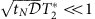, where is the diffusion rate of ΔBz. For an estimation of ΔBz with N different measurements, we generate a random walk of N different values for ΔBz (using the measured diffusion), simulate the outcome of each measurement, and compute the Bayesian estimate of ΔBz using the simulated outcomes. By repeating this procedure 4,096 times, and using the mean squared error, MSE=‹(ΔBz−ΔBzestimated)2› as a metric for performance, we can find the optimal number of measurements to perform. To include the entire error budget of the FPGA apparatus, we add to this MSE the error from the phase noise of the VCO, the measured voltage noise on the analogue output controlling the VCO, and the diffusion of ΔBz during the ‘operation’ period of the experiment.

### Software post-processing

The estimate of ΔBz can be independently verified using software analysis. In this experiment, we use the same method to estimate ΔBz as in the adaptive control experiment, but in the operation segment perform oscillations around ΔBz for verification. We choose m different evolution times and measure each n times for a total of Ntot=m × n measurements of ΔBz. In the ith experiment (i=1,2,…Ntot), we evolve for a time tevo,i, accumulating phase φiBz,itevo,i. Because we make a precise measurement of ΔBz at the start of each experiment, we can employ it to rescale the time, tevo,i, so that the phase accumulated for a given time is constant using the equation,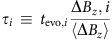This sets φi(τi)=‹ΔBzτi, with residual error arising from inaccuracy in the estimate of ΔBz,i. The data are then sorted by τ, and points of similar τ are averaged using a Gaussian window with στ=0.5 nsT≈16 ns, where T is the period of the oscillations.

How to cite this article: Shulman, M. D. et al. Suppressing qubit dephasing using real-time Hamiltonian estimation. Nat. Commun. 5:5156 doi: 10.1038/ncomms6156 (2014).

## References

1. Wiseman, Howard M. & Milburn, Gerard J. Quantum Measurement and Control Cambridge Univ. Press (2010).

2. Waldherr, G. et al. High-dynamic-range magnetometry with a single nuclear spin in diamond. Nat. Nanotechnol. 7, 105–108 (2012).

3. Nusran, N. M., Ummal, M. & Gurudev Dutt, M. V. High dynamic range magnetometry with a single electronic spin in diamond. Nat. Nanotechnol. 7, 109–113 (2012).

4. Petta, J. R. et al. Coherent manipulation of coupled electron spins in semiconductor quantum dots. Science 309, 2180–2184 (2005).

5. Maune, B. M. et al. Coherent singlet-triplet oscillations in a silicon-based double quantum dot. Nature 481, 344–347 (2011).

6. Dial, O. E. et al. Charge noise spectroscopy using coherent exchange oscillations in a singlet-triplet qubit. Phys. Rev. Lett. 110, 146804 (2013).

7. Shulman, M. D. et al. Demonstration of entanglement of electrostatically coupled singlet-triplet qubits. Science 336, 202–205 (2012).

8. Barthel, C., Reilly, D. J., Marcus, C. M., Hanson, M. P. & Gossard., A. C. Rapid single-shot measurement of a singlet-triplet qubit. Phys. Rev. Lett. 103, 160503 (2009).

9. Reilly, D. J., Marcus, C. M., Hanson, M. P. & Gossard, A. C. Fast single-charge sensing with a RF quantum point contact. Appl. Phys. Lett. 91, 162101 (2007).

10. Foletti, S., Bluhm, H., Mahalu, D., Umansky, V. & Yacoby, A. Universal quantum control in two-electron spin quantum bits using dynamic nuclear polarization. Nat. Phys. 5, 903–908 (2009).

11. Bluhm, H., Foletti, S., Mahalu, D., Umansky, V. & Yacoby, A. Enhancing the coherence of a spin qubit by operating it as a feedback loop that controls its nuclear spin bath. Phys. Rev. Lett. 105, 216803 (2010).

12. Bluhm, H. et al. Dephasing time of GaAs electron-spin qubits coupled to a nuclear bath exceeding 200 μs. Nat. Phys. 7, 109–113 (2011).

13. Sergeevich, A., Chandran, A., Combes, J., Bartlett, S. D. & Wiseman, H. M. Characterization of a qubit Hamiltonian using adaptive measurements in a fixed basis. Phys. Rev. A 84, 052315 (2011).

14. Kestner, J. P., Wang, X., Bishop, L. S., Barnes, E. & Das Sarma, S. Noise-resistant control for a spin qubit array. Phys. Rev. Lett. 110, 140502 (2013).

15. Coish, W. A. & Loss, D. Hyperfine interaction in a quantum dot: non-Markovian electron spin dynamics. Phys. Rev. B 70, 195340 (2004).

16. Klauser, D., Coish, W. A. & Loss, D. Nuclear spin state narrowing via gate-controlled Rabi oscillations in a double quantum dot. Phys. Rev. B 73, 205302 (2006).

17. Ferrie, C., Granade, C. E. & Cory, D. G. How to best sample a periodic probability distribution, or on the accuracy of Hamiltonian finding strategies. Quantum Inf. Process. 12, 611 (2013).

18. Schroer, M. D. & Petta, J. R. Quantum dots: time to get the nukes out. Nat. Phys. 4, 516–518 (2008).

19. Balasubramanian, G. et al. Ultralong spin coherence time in isotopically engineered diamond. Nat. Mater. 8, 383–387 (2009).

20. Wild, A. et al. Few electron double quantum dot in an isotopically purified 28Si quantum wel. Appl. Phys. Lett. 100, 143110 (2012).

21. Muhonen, J. T. et al. Storing quantum information for 30 seconds in a nanoelectronic device. Preprint at http://arxiv.org/abs/1402.7140 (2014).

22. Hahn, E. L. Spin echoes. Phys. Rev. 80, 580–594 (1950).

23. Uhrig, G. S. Exact results on dynamical decoupling by pi pulses in quantum inforamtion processes. New J. Phys. 10, 083024 (2008).

24. Nielsen, M. A. & Chuang, I. L. Quantum Computation and Quantum Information Cambridge Univ. Press (2000).

25. Reed, M. D. et al. Realization of a three-qubit quantum error correction with superconducting circuits. Nature 482, 382–385 (2011).

26. Waldherr, G. et al. Quantum error correction in a solid-state hybrid spin register. Nature 506, 204–207 (2014).

27. Taminiau, T. H., Cramer, J., Van der Sar, T., Dobrovitski, V. V. & Hanson, R. Universal control and error correction in multi-qubit spin registers in diamond. Nat. Nanotechnol. 9, 171–176 (2014).

## Acknowledgements

We acknowledge James MacArthur for building the correlated double sampler. This research was funded by the United States Department of Defense, the Office of the Director of National Intelligence (ODNI), Intelligence Advanced Research Projects Activity (IARPA), and the Army Research Office grant (W911NF-11-1-0068 and W911NF-11-1-0068). All statements of fact, opinion or conclusions contained herein are those of the authors and should not be construed as representing the official views or policies either expressly or implied of of IARPA, the ODNI, or the U.S. Government. S.P.H was supported by the Department of Defense (DoD) through the National Defense Science & Engineering Graduate Fellowship (NDSEG) Program. A.C.D. acknowledges discussions with Matthew Wadrop regarding extracting diffusion constants. A.C.D. and S.D.B. acknowledge support from the ARC via the Centre of Excellence in Engineering Quantum Systems (EQuS) project number CE110001013. This work was performed in part at the Center for Nanoscale Systems (CNS), a member of the National Nanotechnology Infrastructure Network (NNIN), which is supported by the National Science Foundation under NSF award no. ECS-0335765. CNS is a part of Harvard University.

## Author information

Authors

### Contributions

V.U. prepared the crystal M.D.S. fabricated the sample, J.M.N. programmed the FPGA, M.D.S, S.P.H., J.M.N., S.D.B, A.C.D, and A.Y. carried out the experiment, analyzed the data, and wrote the paper.

### Corresponding authors

Correspondence to M. D. Shulman or A. Yacoby.

## Ethics declarations

### Competing interests

The authors declare no conflict of interest.

## Supplementary information

### Supplementary Information

Supplementary Figures 1-4 and Supplementary Notes 1-2 (PDF 868 kb)

## Rights and permissions

Reprints and Permissions

• ### Improving qubit coherence using closed-loop feedback

• Antti Vepsäläinen
• Roni Winik
• William D. Oliver

Nature Communications (2022)

• ### Fast universal quantum gate above the fault-tolerance threshold in silicon

• Akito Noiri
• Kenta Takeda
• Seigo Tarucha

Nature (2022)

• ### Probabilistic teleportation of a quantum dot spin qubit

• Y. Kojima
• T. Nakajima
• S. Tarucha

npj Quantum Information (2021)

• ### A singlet-triplet hole spin qubit in planar Ge

• Daniel Jirovec
• Andrea Hofmann
• Georgios Katsaros

Nature Materials (2021)

• ### Floquet-enhanced spin swaps

• Haifeng Qiao
• John M. Nichol

Nature Communications (2021)